UGC NET December 2013 Paper II solved

UGC NET
COMPUTER SCIENCE & ENGINEERING, DECEMBER 2013
PAPER II
Q1. When data and acknowledgement are sent in the same frame, this is called as
(A) Piggy packing
(B) Piggy backing
(C) Back packing
(D) Good packing
Ans: (B)
Q2. Encryption and Decryption is the responsibility of _______ Layer.
(A) Physical
(B) Network
(C) Application
Ans: (C)
Q3. An analog signal carries 4 bits in each signal unit. If 1000 signal units are sent per second, then baud rate and bit rate of the signal are _______ and _______.
(A) 4000 bauds \ sec & 1000 bps
(B) 2000 bauds \ sec & 1000 bps
(C) 1000 bauds \ sec & 500 bps
(D) 1000 bauds \ sec & 4000 bps
Ans: (D)
Explanation: Baud rate = 1000 bauds per second. Bit rate=1000X4= 4000 bps.
Q4. The VLF and LF bauds use _______ propagation for communication.
(A) Ground
(B) Sky
(C) Line of sight
(D) Space
Ans: (A)
Explanation: There are three types of propagation for communication. 1. Ground propagation, 2. Sky propagation, 3. Line-of-sight propagation. Low frequency signals of Radio waves travel in all directions from the transmitting antenna through the lowest portion of the atmosphere, in Ground propagation.
Q5. Using the RSA public key crypto system, if p = 13, q = 31 and d = 7, then the value of e is
(A) 101
(B) 103
(C) 105
(D) 107
Ans: (B)
Q6. FAN IN of a component A is defined as
(A) Number of components that can call or pass control to component A.
(B) Number of components that are called by component A.
(C) Number of components related to component A.
(D) Number of components dependent on component A.
Ans: (A)
Q7. The relationship of data elements in a module is called
(A) Coupling
(B) Modularity
(C) Cohesion
(D) Granularity
Ans: (C)
Q8. Software Configuration Management is the discipline for systematically controlling
(A) the changes due to the evolution of work products as the project proceeds.
(B) the changes due to defects (bugs) being found and then fixed.
(C) the changes due to requirement changes
(D) all of the above
Ans: (D)

Q9. Which one of the following is not a step of requirement engineering?
(A) Requirement elicitation
(B) Requirement analysis
(C) Requirement design
(D) Requirement documentation
Ans: (C)
Q10. Testing of software with actual data and in actual environment is called
(A) Alpha testing
(B) Beta testing
(C) Regression testing
(D) None of the above
Ans: (B)
Q11. The student marks should not be greater than 100. This is
(A) Integrity constraint
(B) Referential constraint
(C) Over-defined constraint
(D) Feasible constraint
Ans: (A)
Q12. GO BOTTOM and SKIP-3 commands are given one after another in a database file of 30 records. It shifts the control to
(A) 28th record
(B) 27th record
(C) 3rd record
(D) 4th record
Ans: (A)
Q13. An ER Model includes
I. An ER diagram portraying entity types.
II. Attributes for each entity type
III. Relationships among entity types.
IV. Semantic integrity constraints that reflects the business rules about data not captured in the ER diagram.
(A) I, II, III & IV
(B) I & IV
(C) I, II & IV
(D) I & III
Ans: (A)
Q14. Based on the cardinality ratio and participation ________ associated with a relationship type, choose either the Foreign Key Design, the Cross Referencing Design or Mutual Referencing Design.
(A) Entity
(B) Constraints
(C) Rules
(D) Keys
Ans: (B)
Q15. Data Integrity control uses _______
(A) Upper and lower limits on numeric data.
(C) Data dictionary to keep the data
(D) Data dictionary to find last access of data
Ans: (B)
Q16. What does the following declaration mean?
int (*ptr) ;
(A) ptr is an array of pointers of 10 integers.
(B) ptr is a pointer to an array of 10 integers.
(C) ptr is an array of 10 integers.
(D) none of the above.
Ans: (B)
Q17. Which of the following has compilation error in C?
(A) int n = 32 ;
(B) char ch = 65 ;
(C) float f = (float) 3.2 ;
(D) none of the above
Ans: (D)
Explanation: In option (C) type casting of float is necessary to use it, its rule.
Q18. Which of the following operators cannot be overloaded in C+ +?
(A) ∗
(B) + =
(C) = =
(D) : :
Ans: (D)
Explanation: (: :) is called scope resolution operator, which cannot be overloaded in C.
Q19. _________ allows to create classes which are derived from other classes, so that they automatically include some of its “parent’s” members, plus its own members.
(B) Inheritance
(C) Polymorphism
(D) Encapsulation
Ans: (B)
Q20. The correct way to round off a floating number x to an integer value is
(A) y = (int) (x + 0.5)
(B) y = int (x + 0.5)
(C) y = (int) x + 0.5
(D) y = (int) ((int)x + 0.5)
Ans: (A)
Q21. What is the value of the postfix expression?
a b c d + – ∗ (where a = 8, b = 4, c = 2 and d = 5)
(A) –3/8
(B) –8/3
(C) 24
(D) –24
Ans: (D)
Q22. If the queue is implemented with a linked list, keeping track of a front pointer and a rear pointer, which of these pointers will change during an insertion into a non-empty queue?
(A) Neither of the pointers change
(B) Only front pointer changes
(C) Only rear pointer changes
(D) Both of the pointers changes
Ans: (C)
Q23. _______ is often used to prove the correctness of a recursive function.
(A) Diagonalization
(B) Communitivity
(C) Mathematical Induction
(D) Matrix Multiplication
Ans: (C)
Q24. For any B-tree of minimum degree t ≥ 2, every node other than the root must have atleast ________ keys and every node can have at most ________ keys.
(A) t – 1, 2t + 1
(B) t + 1, 2t + 1
(C) t – 1, 2t – 1
(D) t + 1, 2t – 1
Ans: (C)
Q25. Given two sorted list of size ‘m’ and ‘n’ respectively. The number of comparison needed in the worst case by the merge sort algorithm will be
(A) m × n
(B) max (m, n)
(C) min (m, n)
(D) m + n – 1
Ans: (D)
Q26. Given the following statements:
S1: SLR uses follow information to guide reductions. In case of LR and LALR parsers, the look-aheads are associated with the items and they make use of the left context available to the parser.
S2: LR grammar is a larger subclass of context free grammar as compared to that SLR and LALR grammars.
Which of the following is true?
(A) S1 is not correct and S2 is not correct.
(B) S1 is not correct and S2 is correct.
(C) S1 is correct and S2 is not correct.
(D) S1 is correct and S2 is correct.
Ans: (D)
Q27. The context free grammar for the language
L = {an bm | n ≤ m + 3, n ≥ 0, m ≥ 0} is
(A) S → aaa A; A → aAb | B, B → Bb | λ
(B) S → aaaA|λ, A → aAb | B, B → Bb | λ
(C) S → aaaA | aa A | λ, A → aAb | B, B → Bb| λ
(D) S → aaaA | aa A | aA | λ, A → aAb | B, B → Bb | λ
Ans: (D)
Q28. Given the following statements:
S1: If L is a regular language then the language {uv | u ∈ L, v ∈ LR} is also regular.
S2: L = {wwR} is regular language.
Which of the following is true?
(A) S1 is not correct and S2 is not correct.
(B) S1 is not correct and S2 is correct.
(C) S1 is correct and S2 is not correct.
(D) S1 is correct and S2 is correct.
Ans: (C)
Q29. The process of assigning load addresses to the various parts of the program and adjusting the code and data in the program to reflect the assigned addresses is called _______.
(A) Symbol resolution
(B) Parsing
(C) Assembly
(D) Relocation
Ans: (D)
Q30. Which of the following derivations does a top-down parser use while parsing an input string? The input is scanned from left to right.
(A) Leftmost derivation
(B) Leftmost derivation traced out in reverse
(C) Rightmost derivation traced out in reverse
(D) Rightmost derivation
Ans: (A)
Q31. The dual of a Boolean expression is obtained by interchanging
(A) Boolean sums and Boolean products
(B) Boolean sums and Boolean products or interchanging 0’s and 1’s
(C) Boolean sums and Boolean products and interchanging 0’s & 1’s
(D) Interchanging 0’s and 1’s
Ans: (C)
Q32. Given that (292)10 = (1204)x in some number system x. The base x of that number system is
(A) 2
(B) 8
(C) 10
(D) None of the above
Ans: (D)
Q33. The sum of products expansion for the function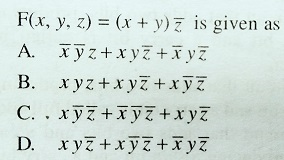Ans: (D)
Q34. Let P(m, n) be the statement
“m divides n” where the universe of discourse for both the variables is the set of positive integers. Determine the truth values of each of the following propositions:
I. ∀m ∀n P(m, n),
II. ∃m ∀n P(m, n)
(A) Both I and II are true
(B) Both I and II are false
(C) I – false & II – true
(D) I – true & II – false
Ans: (C)
Q35. Big – O estimate for
f(x) = (x + 1) log(x2 + 1) + 3x2 is given as
(A) O(x log x)
(B) O(x2)
(C) O(x3)
(D) O(x2 log x)
Ans: (B)
Q36. How many edges are there in a forest of t-trees containing a total of n vertices?
(A) n + t
(B) n – t
(C) n ∗ t
(D) nt
Ans: (B)
Q37. Let f and g be the functions from the set of integers to the set integers defined by
f(x) = 2x + 3 and g(x) = 3x + 2
Then the composition of f and g and g and f is given as
(A) 6x + 7, 6x + 11
(B) 6x + 11, 6x + 7
(C) 5x + 5, 5x + 5
(D) None of the above
Ans: (A)
Q38. If n and r are non-negative integers and n ≥ r, then p(n + 1, r) equals to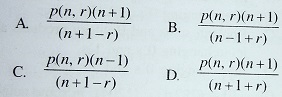Ans: (A)
Q39. A graph is non-planar if and only if it contains a subgraph homomorphic to
(A) K3, 2 or K5
(B) K 3, 3 and K6
(C) K 3, 3 or K5
(D) K2, 3 and K5
Ans: (C)
Q40. Which of the following statements are true?
I. A circuit that adds two bits, producing a sum bit and a carry bit is called half adder.
II. A circuit that adds two bits, producing a sum bit and a carry bit is called full adder.
III. A circuit that adds two bits and a carry bit producing a sum bit and a carry bit is called full adder.
IV. A device that accepts the value of a Boolean variable as input and produces its complement is called an inverter.
(A) I & II
(B) II & III
(C) I, II, III
(D) I, III & IV
Ans: (D)
Q41. Active X controls are Pentium binary programs that can be embedded in ________
(A) Word pages
(B) URL pages
(C) Script pages
(D) Web pages
Ans: (D)
Q42. Match the following:
 List-I List-II a. Wireless Application Environment i. HTTP b. Wireless Transaction Protocol ii. IP c. Wireless Datagram Protocol iii. Scripts d. Wireless iv. UDP
Codes:
 a b C D (A) ii iv I iii (B) iv iii Ii i (C) iv iii I ii (D) iii I Iv ii
Ans: (D)
Q43. Which of the following is widely used inside the telephone system for long-haul data traffic?
(A) ISDN
(B) ATM
(C) Frame Relay
(D) ISTN
Ans: (B)
Q44. The document standards for EDI were first developed by large business house during the 1970s and are now under the control of the following standard organisation:
(A) ISO
(B) ANSI
(C) ITU-T
(D) IEEE
Ans: (B)
Q45. Electronic Data Interchange Software consists of the following four layers:
(A) Business application, Internal format conversion, Network translator, EDI envelope
(B) Business application, Internal format conversion, EDI translator, EDI envelope
(C) Application layer, Transport layer, EDI translator, EDI envelope
(D) Application layer, Transport layer, IP layer, EDI envelope
Ans: (B)
Q46. Consider a pre-emptive priority based scheduling algorithm based on dynamically changing priority. Larger priority number implies higher priority. When the process is waiting for CPU in the ready queue (but not yet started execution), its priority changes at a rate a = 2. When it starts running, its priority changes at a rate b = 1. All the processes are assigned priority value 0 when they enter ready queue. Assume that the following processes want to execute:
 Process ID Arrival Time Service Time P1 0 4 P2 1 1 P3 2 2 P4 3 1
The time quantum q = 1. When two processes want to join ready queue simultaneously, the process which has not executed recently is given priority. The finish time of processes P1, P2, P3 and P4 will respectively be
(A) 4, 5, 7 and 8
(B) 8, 2, 7 and 5
(C) 2, 5, 7 and 8
(D) 8, 2, 5 and 7
Ans: (B)
Explanation: When priority changes at a rate a=2, b=1, Gantt Chart :
So from above Gantt Chart,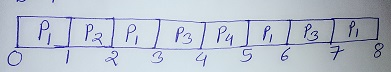Finish time of P1=8,P2=2,P3=7,P4=5.
If we do not consider priority changes than Gantt Chart become: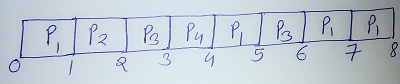So from above Gantt Chart,
Finish time of P1=8,P2=2,P3=6,P4=4.
Q47. The virtual address generated by a CPU is 32 bits. The Translation Look-aside Buffer (TLB) can hold total 64 page table entries and a 4-way set associative (i.e. with 4-cache lines in the set). The page size is 4 KB. The minimum size of TLB tag is
(A) 12 bits
(B) 15 bits
(C) 16 bits
(D) 20 bits
Ans: (C)
Q48. Consider a disk queue with request for input/output to block on cylinders
98, 183, 37, 122, 14, 124, 65, 67
in that order. Assume that disk head is initially positioned at cylinder 53 and moving towards cylinder number 0. The total number of head movements using Shortest Seek Time First (SSTF) and SCAN algorithms are respectively
(A) 236 and 252 cylinders
(B) 640 and 236 cylinders
(C) 235 and 640 cylinders
(D) 235 and 252 cylinders
Ans: (Current ans. is 208 & 236 which not given in option)
Explanation:
SSTF Algorithm when movement to 0 given: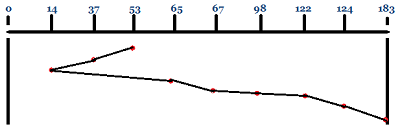SCAN Algorithm: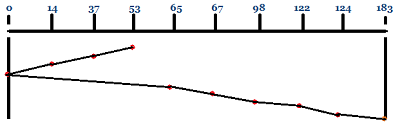SSTF Algorithm when movement to 0 not given: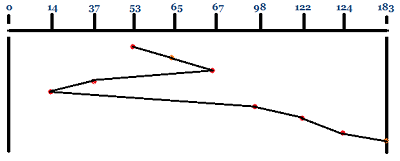Q49. How much space will be required to store the bit map of a 1.3 GB disk with 512 bytes block size?
(A) 332.8 KB
(B) 83.6 KB
(C) 266.2 KB
(D) 256.6 KB
Ans: (A)
Q50. Linux operating system uses
(A) Affinity Scheduling
(B) Fair Preemptive Scheduling
(C) Hand Shaking
(D) Highest Penalty Ratio Next
Ans: (B)